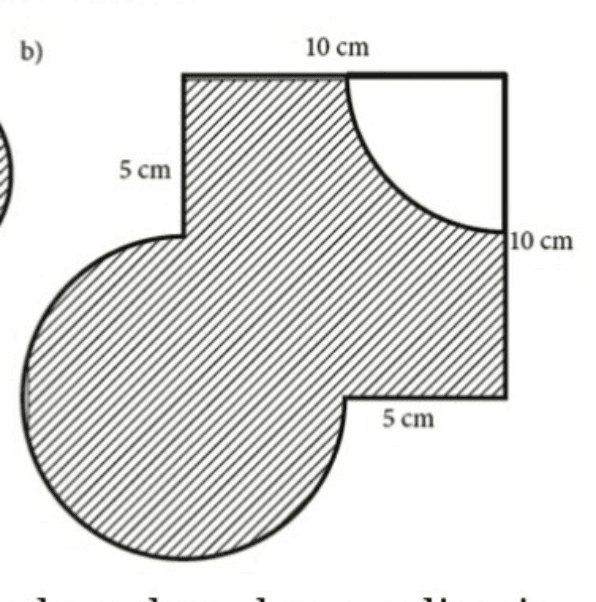# QuestionCan someone assist me to find perimeter and area shaded part this figure. Thanks

The repeated shaded area between the circle and the square is a 1/4 circle.

Hence the shaded area = Area of the square – Area of the 1/4 circle – Area of the 1/4 circle + Area of the circle

Shaded area = Area of the square + Area of the 1/2 circle

= 10² + 1/2 × 5²π

Assume π = 3.14

Shaded area = 100 + 39.25 = 139.25 cm²